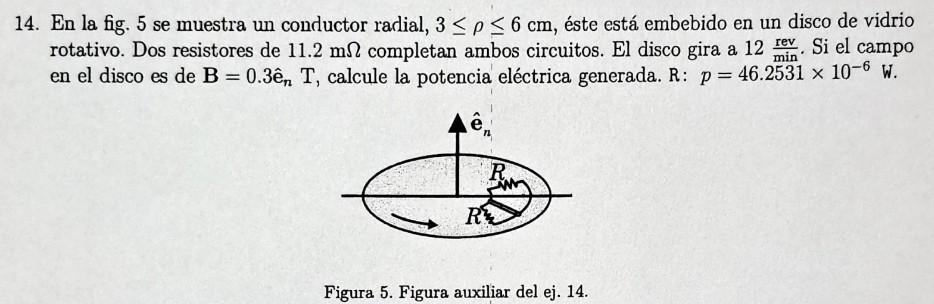# (Solved): 14. In fig. 5 shows a radial conductor, 3 p 6 cm, it is embedded in a rotating glass disk. Two ...

14. In fig. 5 shows a radial conductor, 3 p≤ 6 cm, it is embedded in a rotating glass disk. Two 11.2 m resistors complete both circuits. The disk rotates at 12 rev. If the field min' in the disk is B = 0.3ên T, calculate the electric power generated. A: p = 46.2531 x 10-6 W.14. En la fig. 5 se muestra un conductor radial, , éste está embebido en un disco de vidrio rotativo. Dos resistores de completan ambos circuitos. El disco gira a . Si el campo en el disco es de , calcule la potencia eléctrica generada. . Figura 5. Figura auxiliar del ej. 14.

We have an Answer from Expert

To calculate the electric power generated in the given scenario, we need to use the formula for the power generated in an electric circuit, which is given by:

where P is the power, I is the current, and R is the resistance.
First, let's calculate the current flowing through the circuit. The current can be found using Ohm's law:

where V is the voltage and R_total is the total resistance of the circuit.
The voltage can be calculated using the formula for the electromotive force (emf) induced in a rotating conductor:

where B is the magnetic field strength, A is the area of the conductor, ? is the angular velocity, and ? is the angle between the normal to the conductor's plane and the magnetic field.

We have an Answer from Expert